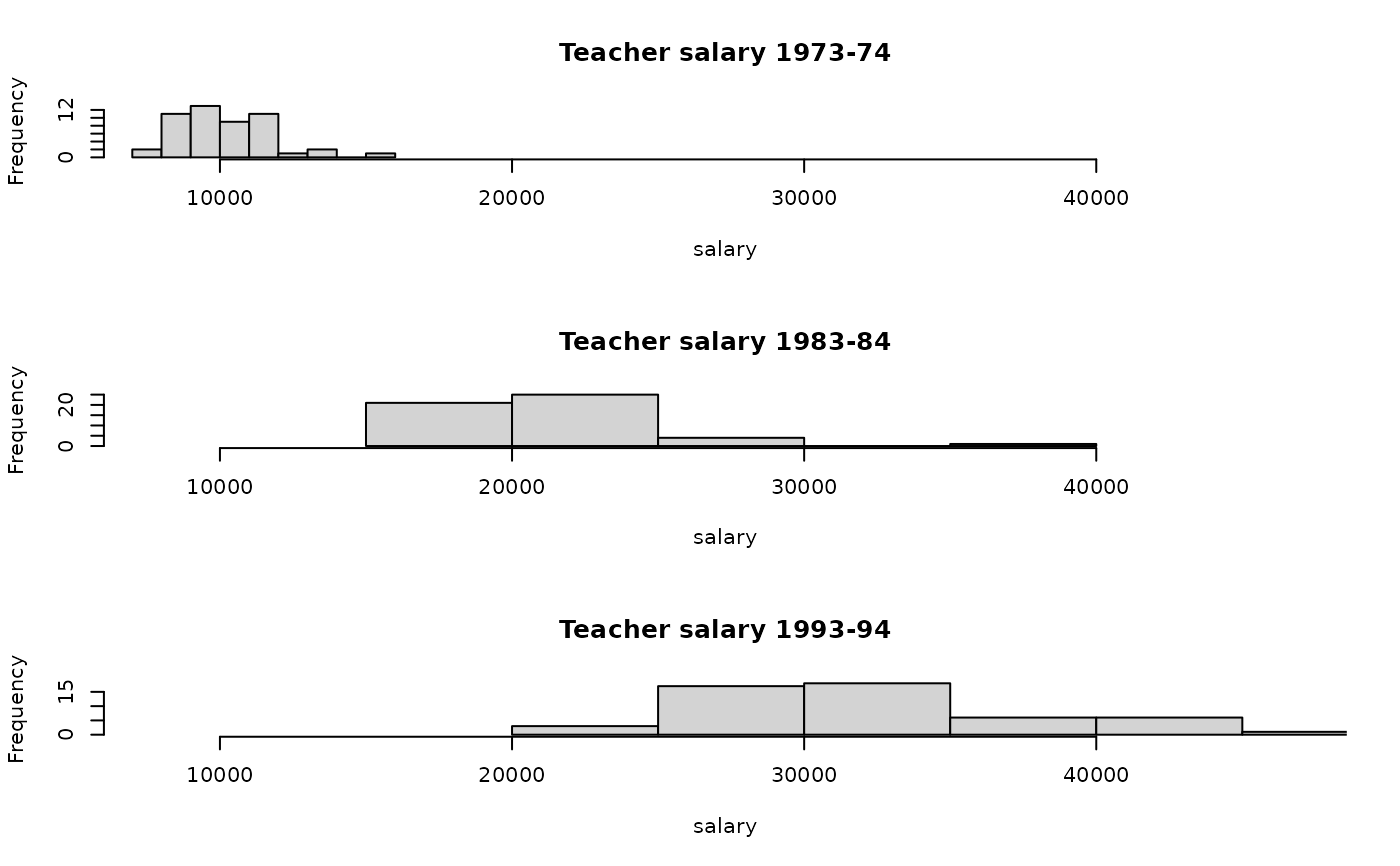Data for Exercise 5.114

Teacher

## Format

A data frame/tibble with 51 observations on three variables

state

U.S. state

year

salary

avaerage salary (in dollars)

## Source

National Education Association.

## Examples


par(mfrow = c(3, 1))
hist(Teacher$salary[Teacher$year == "1973-74"],
main = "Teacher salary 1973-74", xlab = "salary",
xlim = range(Teacher$salary, na.rm = TRUE)) hist(Teacher$salary[Teacher$year == "1983-84"], main = "Teacher salary 1983-84", xlab = "salary", xlim = range(Teacher$salary, na.rm = TRUE))
hist(Teacher$salary[Teacher$year == "1993-94"],
main = "Teacher salary 1993-94", xlab = "salary",
xlim = range(Teacher\$salary, na.rm = TRUE))par(mfrow = c(1, 1))
if (FALSE) {
library(ggplot2)
ggplot2::ggplot(data = Teacher, aes(x = salary)) +
geom_histogram(fill = "purple", color = "black") +
facet_grid(year ~ .) +
theme_bw()
}